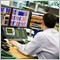# OnTick critical error - backtest - page 2181

Drazen Penic:

You created handles for the H4 and current (presumably not H4) timeframe.

• your code calls GetIndicator() with value of the index variable set to "current" and "current+1"
• variable current is set to 0 and I didn't find any place where it is modified
• that means that you read current value on H4 timeframe and value of the previous bar on the current timeframe
• Is that what you want?

• For debugging purposes, print value of the arr[index] before you return it and check if you got the value you expected
• Check that you read all values from the same end - check that you consistently use xxxAsSeries() functions
If you don't plan to modify variable "current", define local variables in functions where you use it. Or define global constant. Anyway, don't use global variable for something that can be defined locally.

Hi Darzen,

thanks for the extensive help!

1) Yes, I check the values from the current and current+1 bar (H1) and the current value of the H4 bar.

2) I checked the values with the print funtion and all the values are a little bit different, but not more than 5 basispoints and it should not make any difference for the EA signal!

I reduced my code to the basics and changed the Buy and Sell opening functions...... but still no trade?!

```#include <Trade\Trade.mqh>

int handle1 = 1;
int handle2 = 1;

double = 1,
const string = NULL,
double = 0.0,

bool Sell (
double = 1,
const string = NULL,
double = 0.0,
double = SYMBOL_BID+150,
double = SYMBOL_BID-200,
const string = "Sell");
//+------------------------------------------------------------------+
//| Expert initialization function                                   |
//+------------------------------------------------------------------+
int OnInit()
{

handle1 = iCustom(NULL, PERIOD_H4, "Kalman velocity (mtf)",1,PRICE_CLOSE);
handle2 = iCustom(NULL, PERIOD_CURRENT, "Kalman velocity (mtf)",1,PRICE_CLOSE);

return(0);
}

//+------------------------------------------------------------------+
//| Get Low for specified bar index                                  |
//+------------------------------------------------------------------+
double Low(int index)
{
double arr[];
double low = 0;
ArraySetAsSeries(arr, true);
int copied = CopyLow(Symbol(), PERIOD_CURRENT, 0, Bars(Symbol(), PERIOD_CURRENT), arr);
if (copied>0 && index<copied) low = arr[index];
return (low);
}
//+------------------------------------------------------------------+
//| Get the High for specified bar index                             |
//+------------------------------------------------------------------+
double High(int index)
{
double arr[];
double high = 0;
ArraySetAsSeries(arr, true);
int copied = CopyHigh(Symbol(), PERIOD_CURRENT, 0, Bars(Symbol(), PERIOD_CURRENT), arr);
if (copied>0 && index<copied) high=arr[index];
return(high);
}
//+------------------------------------------------------------------+
//| Get Close for specified bar index                                |
//+------------------------------------------------------------------+
double Close(int index)
{
double arr[];
double close = 0;
ArraySetAsSeries(arr, true);
int copied = CopyClose(Symbol(), PERIOD_CURRENT, 0, Bars(Symbol(), PERIOD_CURRENT), arr);
if (copied>0 && index<copied) close = arr[index];
return (close);
}
//+------------------------------------------------------------------+
//| Get Open for specified bar index                                 |
//+------------------------------------------------------------------+
double Open(int index)
{
double arr[];
double open = 0;
ArraySetAsSeries(arr, true);
int copied = CopyOpen(Symbol(), PERIOD_CURRENT, 0, Bars(Symbol(), PERIOD_CURRENT), arr);
if (copied>0 && index<copied) open = arr[index];
return (open);
}
//+------------------------------------------------------------------+
//| Get current bid value                                            |
//+------------------------------------------------------------------+
double Bid()
{
return (SymbolInfoDouble(Symbol(), SYMBOL_BID));
}

//+------------------------------------------------------------------+
//| Get current ask value                                            |
//+------------------------------------------------------------------+
{
}

//+------------------------------------------------------------------+
//| Get indicator value back                                         |
//+------------------------------------------------------------------+

double GetIndicator(int handle, int buffer_num, int index)
{
//--- array for the indicator values
double arr[];
//--- obtain the indicator value in the last two bars
if (CopyBuffer(handle, buffer_num, 0, index+1, arr) <= 0)
{
Sleep(200);
for(int i=0; i<100; i++)
{
if (BarsCalculated(handle) > 0)
break;
Sleep(50);
}
int copied = CopyBuffer(handle, buffer_num, 0, index+1, arr);
if (copied <= 0)
{
Print("CopyBuffer failed. Maybe history has not download yet? Error = ", GetLastError());
return -1;
}
else
Print(arr[index]);

return (arr[index]);
}
else
{
Print(arr[index]);
return (arr[index]);
}

return 0;
}

void TechnicalAnalysis()
{

if (GetIndicator(handle1,0,0) > 0)
{
TechnicalAnalysis2();

}
}

void TechnicalAnalysis2()
{

if ((GetIndicator(handle2,0,0) > 0) && (GetIndicator(handle2,1,1) < 0)&& (PositionsTotal()<1))

}

void TechnicalAnalysis3()
{

if (GetIndicator(handle1,0,0) < 0)
{
TechnicalAnalysis2();

}
}

void TechnicalAnalysis4()
{

if ((GetIndicator(handle2,0,0) < 0) && (GetIndicator(handle2,1,1) > 0)&& (PositionsTotal()<1))
Sell();

}

```3123

Maximilian Goldbecker:

Hi Darzen,

thanks for the extensive help!

1) Yes, I check the values from the current and current+1 bar (H1) and the current value of the H4 bar.

2) I checked the values with the print funtion and all the values are a little bit different, but not more than 5 basispoints and it should not make any difference for the EA signal!

I reduced my code to the basics and changed the Buy and Sell opening functions...... but still no trade?!

If you did debugging then either:

• your strategy does not work or
• you missed something while debugging

If you did debugging then you must know which function did not call the next one.
So, where and why does your chain stop?

For debugging purposes do something like this:

```void TechnicalAnalysis2()
{
double bar0 = GetIndicator(handle2,0,0);
bool bar0Above = bar0 > 0;
double bar1 = GetIndicator(handle2,1,1);
bool bar1Below = bar1 < 0;
bool cross = bar0Above && bar1Below;
bool noPositions = PositionsTotal()<1;

if (cross && noPositions)
{
}
else
{
Print(__FUNCTION__, " STOP! ");
Print(bar0, bar1, PositionTotal());
}

}```

(above code is written directly here in the forum editor, not tested)

Add similar modifications to all functions in the chain and detect where and why it stops.181

Drazen Penic:

If you did debugging then either:

• your strategy does not work or
• you missed something while debugging

If you did debugging then you must know which function did not call the next one.
So, where and why does your chain stop?

For debugging purposes do something like this:

(above code is written directly here in the forum editor, not tested)

Add similar modifications to all functions in the chain and detect where and why it stops.

I tried the debugging again and used your helpfull tips! I think now its working, finally!!! I will check, if the trades and values are fine this week, but it looks good so far. In the end it was a problem with the iCustom function.

Thanks a lot for your help! I really appreiate it!